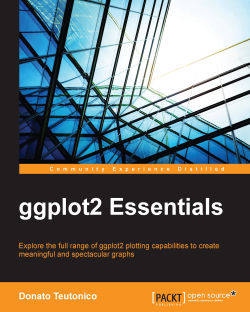# ggplot2 Essentials4.5 (2 reviews total)
By Donato Teutonico
• \$20.99 eBook
• \$25.99 Print + eBook
• \$12.99 eBook + Subscription
What do you get with a Packt Subscription?

• Constantly updated with 100+ new titles each month
• Breadth and depth in over 1,000+ technologies

ggplot2 is one of the most sophisticated and advanced packages of R and its use is constantly growing in the community of R programmers. This book is the perfect starting point for your journey in learning about one of the most refined and widely used plotting tools—ggplot2.

You will start by taking a tour of the most relevant packages available in R to create plots, such as graphics, lattice, and ggplot2. Following this, you will take a deep dive into the concepts of ggplot2 by covering different kinds of plots that will help you gear up for your first hands-on project. After this first taste of the capabilities of ggplot2, you will explore in detail the visualization approach implemented in this package, the so-called grammar of graphics, providing you with the basics to understand the backstage concepts in the plotting functions. Finally, you will take a look at some specialized and sophisticated applications of ggplot2, such as how to realize a complex scatterplot matrix, heatmaps, and how to make spatial visualization graphs.

Publication date:
June 2015
Publisher
Packt
Pages
234
ISBN
9781785283529

## Chapter 1. Graphics in R

The objective of this chapter is to provide you with a general overview of the plotting environments in R and of the most efficient way of coding your graphs in it. We will go through the most important Integrated Development Environment (IDE) available for R as well as the most important packages available for plotting data; this will help you to get an overview of what is available in R and how those packages are compared with `ggplot2`. Finally, we will dig deeper into the grammar of graphics, which represents the basic concepts on which `ggplot2` was designed. But first, let's make sure that you have a working version of R on your computer.

## Getting ggplot2 up and running

If you have this book in your hands, it is very likely you already have a working version of R installed on your computer. If this is not the case, you can download the most up-to-date version of R from the R project website (http://www.r-project.org/). There, you will find a direct connection to the Comprehensive R Archive Network (CRAN), a network of FTP and web servers around the world that store identical, up-to-date versions of code and documentation for R. In addition to access to the CRAN servers, on the website of the R project, you may also find information about R, a few technical manuals, the R journal, and details about the packages developed for R and stored in the CRAN repositories.

At the time of writing, the current version of R is 3.1.2. If you have already installed R on your computer, you can check the actual version with the `R.Version()` code, or for a more concise result, you can use the `R.version.string` code that recalls only part of the output of the previous function.

### Packages in R

In the next few pages of this chapter, we will quickly go through the most important visualization packages available in R, so in order to try the code, you will also need to have additional packages as well as `ggplot2` up and running in your R installation. In the basic R installation, you will already have the `graphics` package available and loaded in the session; the `lattice` package is already available among the standard packages delivered with the basic installation, but it is not loaded by default. `ggplot2`, on the other hand, will need to be installed. You can install and load a package with the following code:

```> install.packages("ggplot2")
> library(ggplot2)
```

Keep in mind that every time R is started, you will need to load the package you need with the `library(name_of_the_package)` command to be able to use the functions contained in the package. In order to get a list of all the packages installed on your computer, you can use the call to the `library()` function without arguments. If, on the other hand, you would like to have a list of the packages currently loaded in the workspace, you can use the `search()` command. One more function that can turn out to be useful when managing your library of packages is `.libPaths()`, which provides you with the location of your R libraries. This function is very useful to trace back the package libraries you are currently using, if any, in addition to the standard library of packages, which on Windows is located by default in a path of the kind `C:/Program Files/R/R-3.1.2/library`.

The following list is a short recap of the functions just discussed:

```.libPaths()   # get library location
library()   # see all the packages installed
search()   # see the packages currently loaded```

## The Integrated Development Environment

You will definitely be able to run the code and the examples shown in this book directly from the standard R Graphical User Interface (GUI), especially if you are frequently working with R in more complex projects or simply if you like to keep an eye on the different components of your code, such as scripts, plots, and help pages, you may well think about the possibility of using an IDE. The number of specific IDEs that get integrated with R is still limited, but some of them are quite efficient, well-designed and open source.

### RStudio

RStudio (http://www.rstudio.com/) is a very nice and advanced programming environment developed specifically for R, and this would be my recommended choice of IDE as the R programming environment in most cases. It is available for all the major platforms (Windows, Linux, and Mac OS X), and it can be run on a local machine, such as your computer, or even over the Web, using RStudio Server. With RStudio Server, you can connect a browser-based interface (the RStudio IDE) to a version of R running on a remote Linux server.

RStudio allows you to integrate several useful functionalities, in particular if you use R for a more complex project. The way the software interface is organized allows you to keep an eye on the different activities you very often deal with in R, such as working on different scripts, overviewing the installed packages, as well as having easy access to the help pages and the plots generated. This last feature is particularly interesting for `ggplot2` since in RStudio, you will be able to easily access the history of the plots created instead of visualizing only the last created plot, as is the case in the default R GUI. One other very useful feature of RStudio is code completion. You can, in fact, start typing a comment, and upon pressing the Tab key, the interface will provide you with functions matching what you have written . This feature will turn out to be very useful in `ggplot2`, so you will not necessarily need to remember all the functions and you will also have guidance for the arguments of the functions as well.

In Figure 1.1, you can see a screenshot from the current version of RStudio (v 0.98.1091):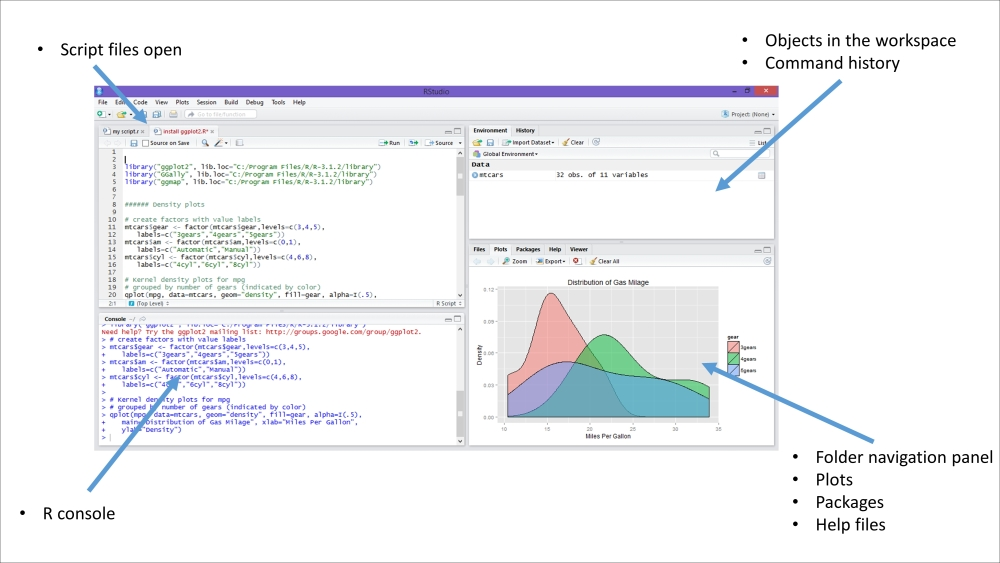Figure 1.1: This is a screenshot of RStudio on Windows 8

The environment is composed of four different areas:

• Scripting area: In this area you can open, create, and write the scripts.

• Console area: This area is the actual R console in which the commands are executed. It is possible to type commands directly here in the console or write them in a script and then run them on the console (I would recommend the last option).

• Workspace/History area: In this area, you can find a practical summary of all the objects created in the workspace in which you are working and the history of the typed commands.

• Visualization area: Here, you can easily load packages, open R help files, and, even more importantly, visualize plots.

The RStudio website provides a lot of material on how to use the program, such as manuals, tutorials, and videos, so if you are interested, refer to the website for more details.

### Eclipse and StatET

Eclipse (http://www.eclipse.org/) is a very powerful IDE that was mainly developed in Java and initially intended for Java programming. Subsequently, several extension packages were also developed to optimize the programming environment for other programming languages, such as C++ and Python. Thanks to its original objective of being a tool for advanced programming, this IDE is particularly intended to deal with very complex programming projects, for instance, if you are working on a big project folder with many different scripts. In these circumstances, Eclipse could help you to keep your programming scripts in order and have easy access to them. One drawback of such a development environment is probably its big size (around 200 MB) and a slightly slow-starting environment.

Eclipse does not support interaction with R natively, so in order to be able to write your code and execute it directly in the R console, you need to add StatET to your basic Eclipse installation. StatET (http://www.walware.de/goto/statet) is a plugin for the Eclipse IDE, and it offers a set of tools for R coding and package building. More detailed information on how to install Eclipse and StatET and how to configure the connections between R and Eclipse/StatET can be found on the websites of the related projects.

### Emacs and ESS

Emacs (http://www.gnu.org/software/emacs/) is a customizable text editor and is very popular, particularly in the Linux environment. Although this text editor appears with a very simple GUI, it is an extremely powerful environment, particularly thanks to the numerous keyboard shortcuts that allow interaction with the environment in a very efficient manner after getting some practice. Also, if the user interface of a typical IDE, such as RStudio, is more sophisticated and advanced, Emacs may be useful if you need to work with R on systems with a poor graphical interface, such as servers and terminal windows. Like Eclipse, Emacs does not support interfacing with R by default, so you will need to install an add-on package on your Emacs that will enable such a connection, Emacs Speaks Statistics (ESS). ESS (http://ess.r-project.org/) is designed to support the editing of scripts and interacting with various statistical analysis programs including R. The objective of the ESS project is to provide efficient text editor support to statistical software, which in some cases comes with a more or less defined GUI, but for which the real power of the language is only accessible through the original scripting language.

## The plotting environments in R

R provides a complete series of options to realize graphics, which makes it quite advanced with regard to data visualization. Along the next few sections of this chapter, we will go through the most important R packages for data visualization by quickly discussing some high-level differences and analogies. If you already have some experience with other R packages for data visualization, in particular `graphics` or `lattice`, the following sections will provide you with some references and examples of how the code used in such packages appears in comparison with that used in `ggplot2`. Moreover, you will also have an idea of the typical layout of the plots created with a certain package, so you will be able to identify the tool used to realize the plots you will come across.

The core of graphics visualization in R is within the `grDevices` package, which provides the basic structure of data plotting, such as the colors and fonts used in the plots. Such a graphic engine was then used as the starting point in the development of more advanced and sophisticated packages for data visualization, the most commonly used being `graphics` and `grid`.

The `graphics` package is often referred to as the base or traditional graphics environment since, historically, it was the first package for data visualization available in R, and it provides functions that allow the generation of complete plots.

The `grid` package, on the other hand, provides an alternative set of graphics tools. This package does not directly provide functions that generate complete plots, so it is not frequently used directly to generate graphics, but it is used in the development of advanced data visualization packages. Among the grid-based packages, the most widely used are `lattice` and `ggplot2`, although they are built by implementing different visualization approaches—`Trellis` plots in the case of `lattice` and the grammar of graphics in the case of `ggplot2`. We will describe these principles in more detail in the coming sections. A diagram representing the connections between the tools just mentioned is shown in Figure 1.2. Just keep in mind that this is not a complete overview of the packages available but simply a small snapshot of the packages we will discuss. Many other packages are built on top of the tools just mentioned, but in the following sections, we will focus on the most relevant packages used in data visualization, namely `graphics`, `lattice`, and, of course, `ggplot2`. If you would like to get a more complete overview of the graphics tools available in R, you can have a look at the web page of the R project summarizing such tools, http://cran.r-project.org/web/views/Graphics.html.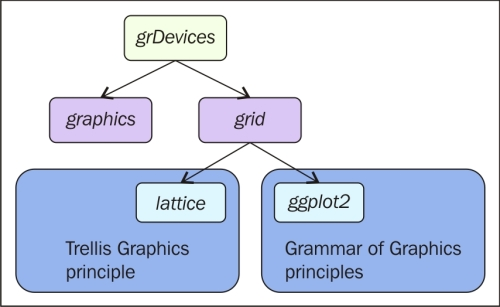Figure 1.2: This is an overview of the most widely used R packages for graphics

In order to see some examples of plots in `graphics`, `lattice` and `ggplot2`, we will go through a few examples of different plots over the following pages. The objective of providing these examples is not to do an exhaustive comparison of the three packages but simply to provide you with a simple comparison of how the different codes as well as the default plot layouts appear for these different plotting tools. For these examples, we will use the `Orange` dataset available in R; to load it in the workspace, simply write the following code:

```>data(Orange)
```

This dataset contains records of the growth of orange trees. You can have a look at the data by recalling its first lines with the following code:

```>head(Orange)
```

You will see that the dataset contains three columns. The first one, `Tree`, is an ID number indicating the tree on which the measurement was taken, while `age` and `circumference` refer to the age in days and the size of the tree in millimeters, respectively. If you want to have more information about this data, you can have a look at the help page of the dataset by typing the following code:

```?Orange
```

Here, you will find the reference of the data as well as a more detailed description of the variables included.

## Standard graphics and grid-based graphics

The existence of these two different graphics environments brings these questions to most users' minds—which package to use and under which circumstances? For simple and basic plots, where the data simply needs to be represented in a standard plot type (such as a scatter plot, histogram, or boxplot) without any additional manipulation, then all the plotting environments are fairly equivalent. In fact, it would probably be possible to produce the same type of plot with `graphics` as well as with `lattice` or `ggplot2`. Nevertheless, in general, the default graphic output of `ggplot2` or `lattice` will be most likely superior compared to `graphics` since both these packages are designed considering the principles of human perception deeply and to make the evaluation of data contained in plots easier.

When more complex data should be analyzed, then the grid-based packages, `lattice` and `ggplot2`, present a more sophisticated support in the analysis of multivariate data. On the other hand, these tools require greater effort to become proficient because of their flexibility and advanced functionalities. In both cases, `lattice` and `ggplot2`, the package provides a full set of tools for data visualization, so you will not need to use `grid` directly in most cases, but you will be able to do all your work directly with one of those packages.

## Graphics and standard plots

The `graphics` package was originally developed based on the experience of the graphics environment in R. The approach implemented in this package is based on the principle of the pen-on-paper model, where the plot is drawn in the first function call and once content is added, it cannot be deleted or modified.

In general, the functions available in this package can be divided into high-level and low-level functions. High-level functions are functions capable of drawing the actual plot, while low-level functions are functions used to add content to a graph that was already created with a high-level function.

### Tip

You can download the example code files from your account at http://www.packtpub.com for all the Packt Publishing books you have purchased. If you purchased this book elsewhere, you can visit http://www.packtpub.com/support and register to have the files e-mailed directly to you.

Let's assume that we would like to have a look at how age is related to the circumference of the trees in our dataset `Orange`; we could simply plot the data on a scatter plot using the high-level function `plot()` as shown in the following code:

```plot(age~circumference, data=Orange)
```

This code creates the graph in Figure 1.3. As you would have noticed, we obtained the graph directly with a call to a function that contains the variables to plot in the form of y~x, and the dataset to locate them. As an alternative, instead of using a formula expression, you can use a direct reference to x and y, using code in the form of `plot(x,y)`. In this case, you will have to use a direct reference to the data instead of using the data argument of the function. Type in the following code:

```plot(Orange\$circumference, Orange\$age)
```

The preceding code results in the following output: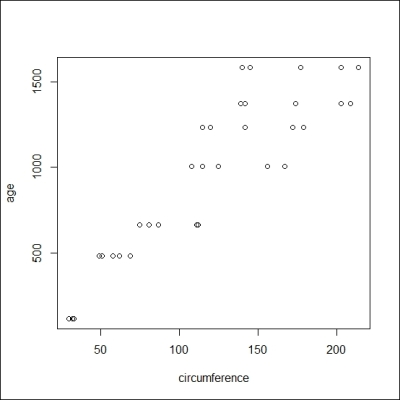Figure 1.3: Simple scatterplot of the dataset Orange using graphics

For the time being, we are not interested in the plot's details, such as the title or the axis, but we will simply focus on how to add elements to the plot we just created. For instance, if we want to include a regression line as well as a smooth line to have an idea of the relation between the data, we should use a low-level function to add the just-created additional lines to the plot; this is done with the `lines()` function:

```plot(age~circumference, data=Orange)   ###Create basic plot
abline(lm(Orange\$age~Orange\$circumference), col="blue")
lines(loess.smooth(Orange\$circumference,Orange\$age), col="red")
```

The graph generated as the output of this code is shown in Figure 1.4: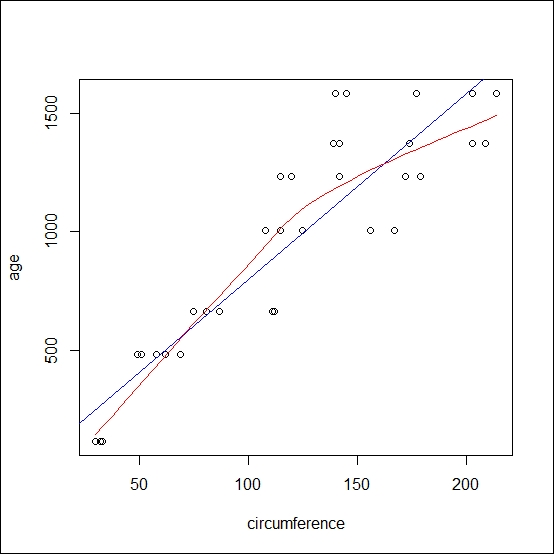Figure 1.4: This is a scatterplot of the Orange data with a regression line (in blue) and a smooth line (in red) realized with graphics

As illustrated, with this package, we have built a graph by first calling one function, which draws the main plot frame, and then additional elements were included using other functions. With graphics, only additional elements can be included in the graph without changing the overall plot frame defined by the `plot()` function. This ability to add several graphical elements together to create a complex plot is one of the fundamental elements of R, and you will notice how all the different graphical packages rely on this principle. If you are interested in getting other code examples of plots in graphics, there is also some demo code available in R for this package, and it can be visualized with `demo(graphics)`.

In the coming sections, you will find a quick reference to how you can generate a similar plot using `graphics` and `ggplot2`. As will be described in more detail later on, in `ggplot2`, there are two main functions to realize plots, `ggplot()` and `qplot()`. The function `qplot()` is a wrapper function that is designed to easily create basic plots with `ggplot2`, and it has a similar code to the `plot()` function of `graphics`. Due to its simplicity, this function is the easiest way to start working with `ggplot2`, so we will use this function in the examples in the following sections. The code in these sections also uses our example dataset `Orange`; in this way, you can run the code directly on your console and see the resulting output.

### Scatterplots with individual data points

To generate the plot generated using `graphics`, use the following code:

```plot(age~circumference, data=Orange)
```

The preceding code results in the following output: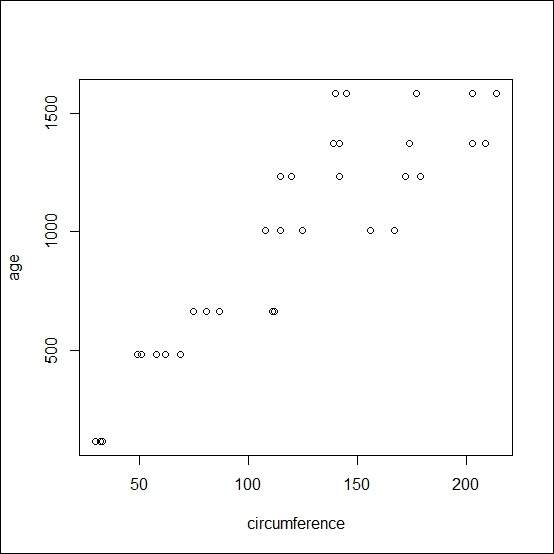To generate the plot using `ggplot2`, use the following code:

```qplot(circumference,age, data=Orange)
```

The preceding code results in the following output: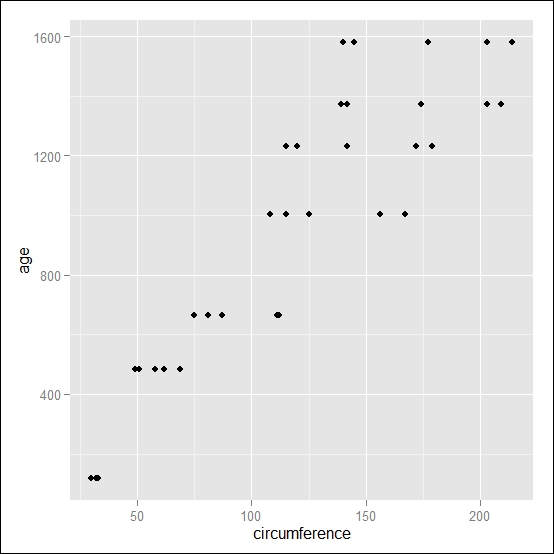### Scatterplots with the line of one tree

To generate the plot using `graphics`, use the following code:

```plot(age~circumference, data=Orange[Orange\$Tree==1,], type="l")
```

The preceding code results in the following output: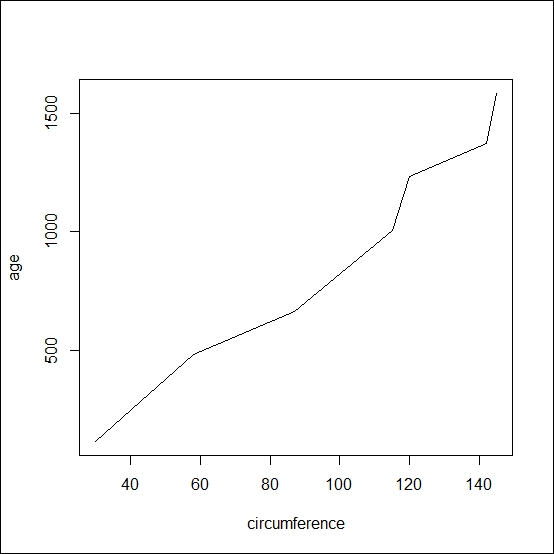To generate the plot using `ggplot2`, use the following code:

```qplot(circumference,age, data=Orange[Orange\$Tree==1,], geom="line")
```

The preceding code results in the following output: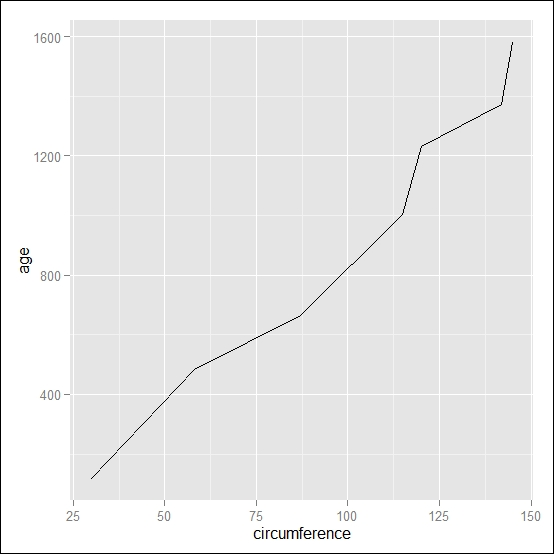### Scatterplots with the line and points of one tree

To generate the plot using `graphics`, use the following code:

```plot(age~circumference, data=Orange[Orange\$Tree==1,], type="b")
```

The preceding code results in the following output: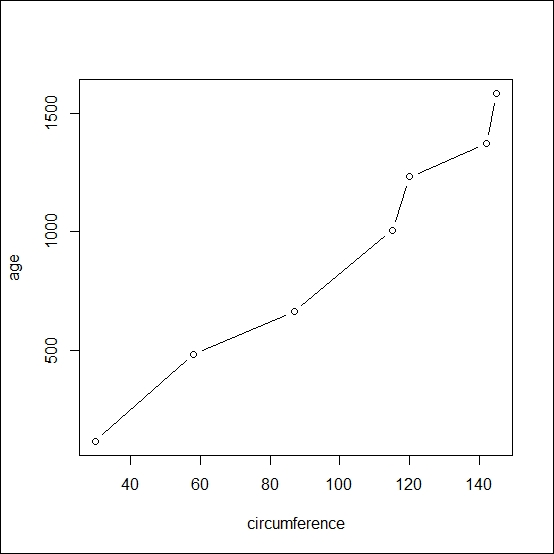To generate the plot using `ggplot2`, use the following code:

```qplot(circumference,age, data=Orange[Orange\$Tree==1,], geom=c("line","point"))
```

The preceding code results in the following output: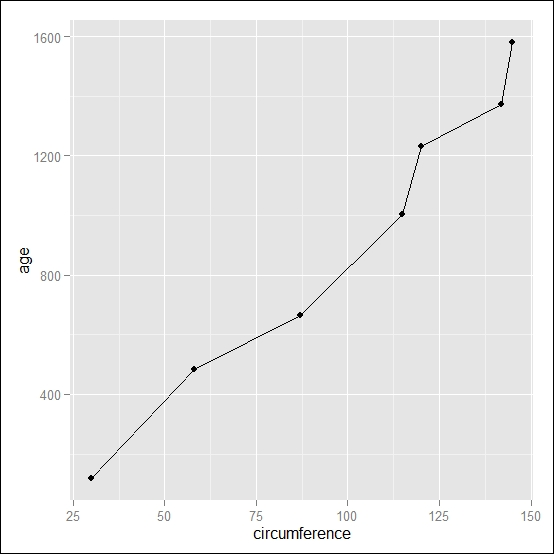### Boxplots of the orange dataset

To generate the plot using `graphics`, use the following code:

```boxplot(circumference~Tree, data=Orange)
```

The preceding code results in the following output: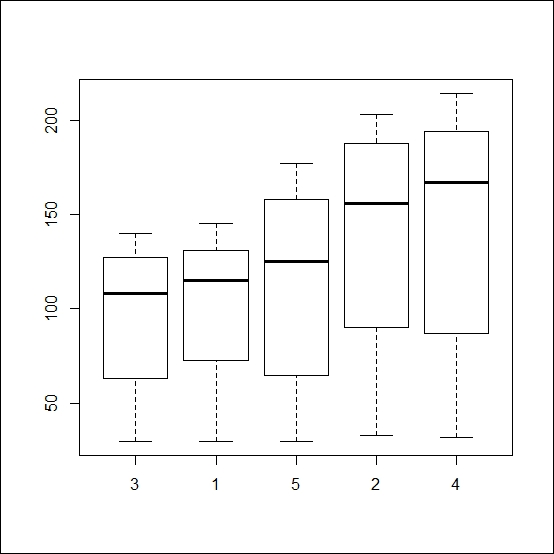To generate the plot using `ggplot2`, use the following code:

```qplot(Tree,circumference, data=Orange, geom="boxplot")
```

The preceding code results in the following output: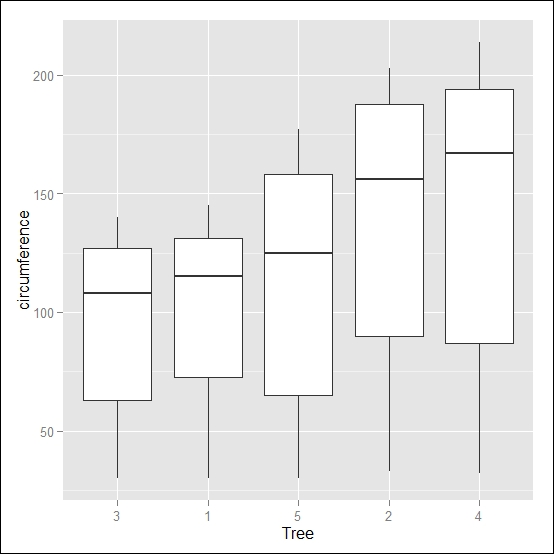### Boxplots with individual observations

To generate the plot using `graphics`, use the following code:

```boxplot(circumference~Tree, data=Orange)
points(circumference~Tree, data=Orange)
```

The preceding code results in the following output: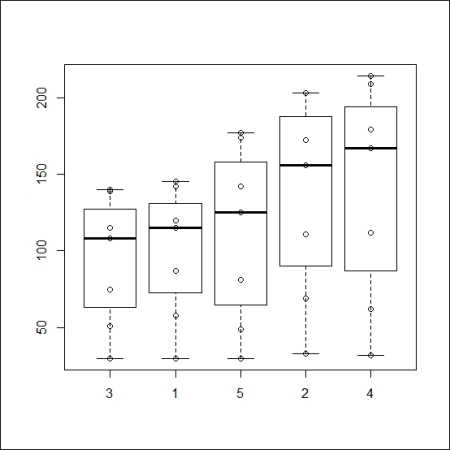To generate the plot using `ggplot2`, use the following code:

```qplot(Tree,circumference, data=Orange, geom=c("boxplot","point"))
```

The preceding code results in the following output: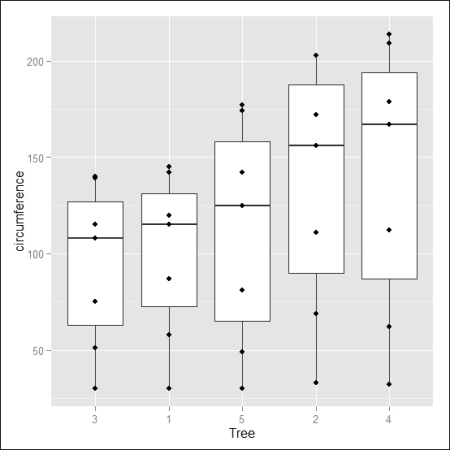### Histograms of the orange dataset

To generate the plot using `graphics`, use the following code:

```hist(Orange\$circumference)
```

The preceding code results in the following output: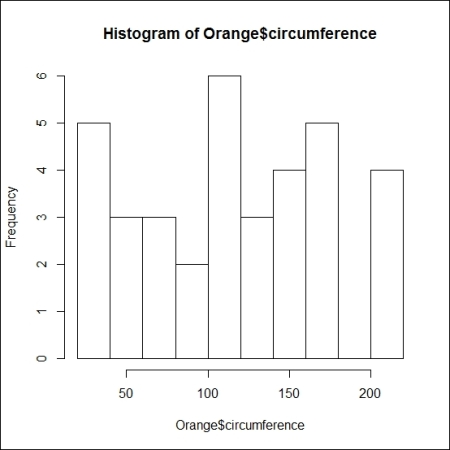To generate the plot using `ggplot2`, use the following code:

```qplot(circumference, data=Orange, geom="histogram")
```

The preceding code results in the following output: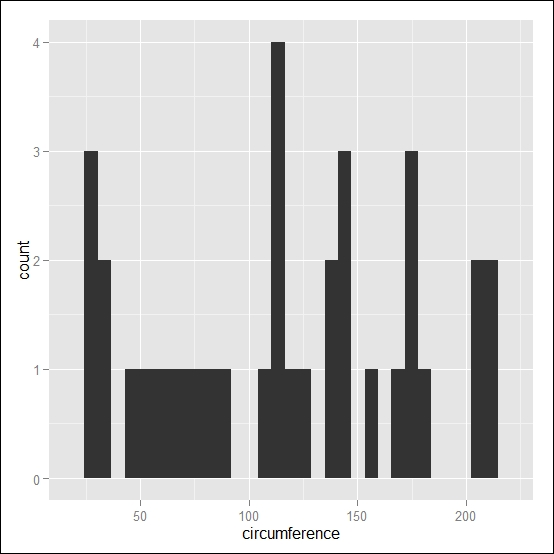### Histograms with the reference line at the median value in red

To generate the plot using `graphics`, use the following code:

```hist(Orange\$circumference)
abline(v=median(Orange\$circumference), col="red")
```

The preceding code results in the following output: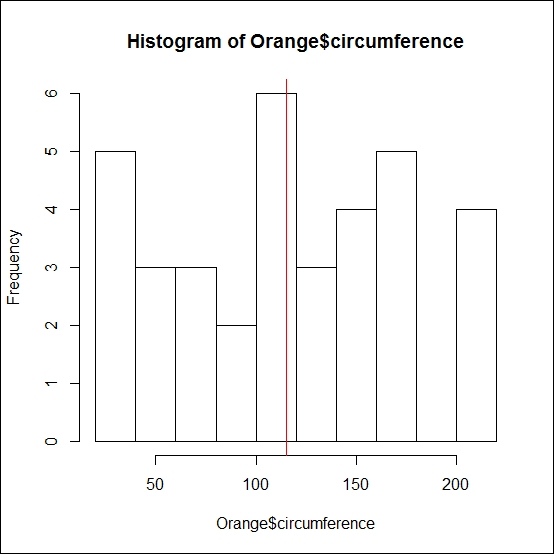To generate the plot using `ggplot2`, use the following code:

```qplot(circumference, data=Orange, geom="histogram")+geom_vline(xintercept = median(Orange\$circumference), colour="red")
```

The preceding code results in the following output: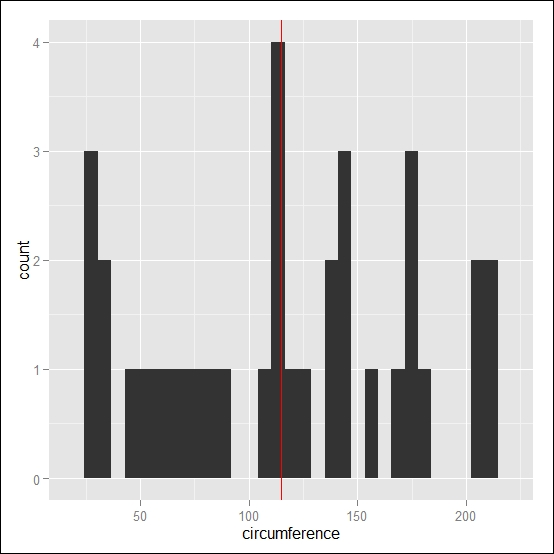## Lattice and Trellis plots

Along with with `graphics`, the base R installation also includes the `lattice` package. This package implements a family of techniques known as Trellis graphics, proposed by William Cleveland to visualize complex datasets with multiple variables. The objective of those design principles was to ensure the accurate and faithful communication of data information. These principles are embedded into the package and are already evident in the default plot design settings. One interesting feature of Trellis plots is the option of multipanel conditioning, which creates multiple plots by splitting the data on the basis of one variable. A similar option is also available in `ggplot2`, but in that case, it is called faceting.

In `lattice`, we also have functions that are able to generate a plot with one single call, but once the plot is drawn, it is already final. Consequently, plot details as well as additional elements that need to be included in the graph, need to be specified already within the call to the main function. This is done by including all the specifications in the panel function argument. These specifications can be included directly in the main body of the function or specified in an independent function, which is then called; this last option usually generates more readable code, so this will be the approach used in the following examples. For instance, if we want to draw the same plot we just generated in the previous section with graphics, containing the age and circumference of trees and also the regression and smooth lines, we need to specify such elements within the function call. You may see an example of the code here; remember that `lattice` needs to be loaded in the workspace:

```require(lattice)              ##Load lattice if needed
myPanel <- function(x,y){
panel.loess(x,y,col="red")      # Add the smooth line
}
xyplot(age~circumference, data=Orange, panel=myPanel)
```

This code produces the plot in Figure 1.5: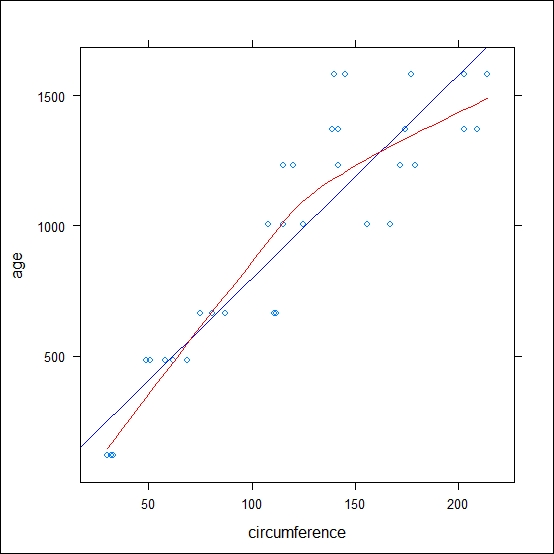Figure 1.5: This is a scatter plot of the Orange data with the regression line (in blue) and the smooth line (in red) realized with lattice

As you would have noticed, taking aside the code differences, the plot generated does not look very different from the one obtained with `graphics`. This is because we are not using any special visualization feature of `lattice`. As mentioned earlier, with this package, we have the option of multipanel conditioning, so let's take a look at this. Let's assume that we want to have the same plot but for the different trees in the dataset. Of course, in this case, you would not need the regression or the smooth line, since there will only be one tree in each plot window, but it could be nice to have the different observations connected. This is shown in the following code:

```myPanel <- function(x,y){
panel.xyplot(x,y, type="b") #the observations
}
xyplot(age~circumference | Tree, data=Orange, panel=myPanel)
```

This code generates the graph shown in Figure 1.6: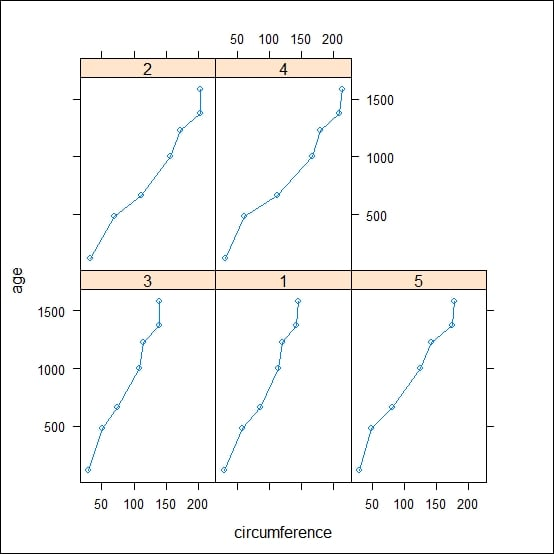Figure 1.6: This is a scatterplot of the Orange data realized with lattice, with one subpanel representing the individual data of each tree. The number of trees in each panel is reported in the upper part of the plot area

As illustrated, using the vertical bar `|`, we are able to obtain the plot conditional to the value of the variable `Tree`. In the upper part of the panels, you would notice the reference to the value of the conditional variable, which, in this case, is the column `Tree`. As mentioned before, `ggplot2` offers this option too; we will see one example of that in the next section.

In the next section, You would find a quick reference to how to convert a typical plot type from `lattice` to `ggplot2`. In this case, the examples are adapted to the typical plotting style of the `lattice` plots.

### Scatterplots with individual observations

To plot the graph using `lattice`, use the following code:

```xyplot(age~circumference, data=Orange)
```

The preceding code results in the following output: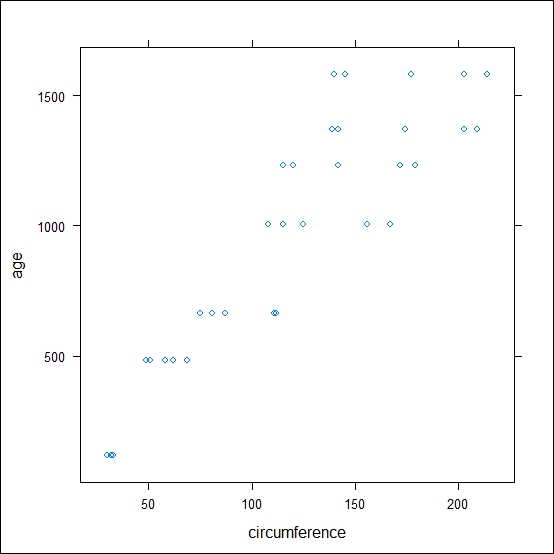To plot the graph using `ggplot2`, use the following code:

```qplot(circumference,age, data=Orange)
```

The preceding code results in the following output:### Scatterplots of the orange dataset with faceting

To plot the graph using `lattice`, use the following code:

```xyplot(age~circumference|Tree, data=Orange)
```

The preceding code results in the following output: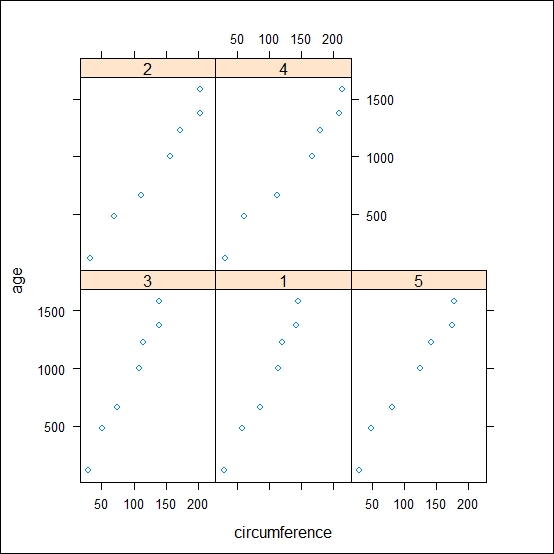To plot the graph using `ggplot2`, use the following code:

```qplot(circumference,age, data=Orange, facets=~Tree)
```

The preceding code results in the following output: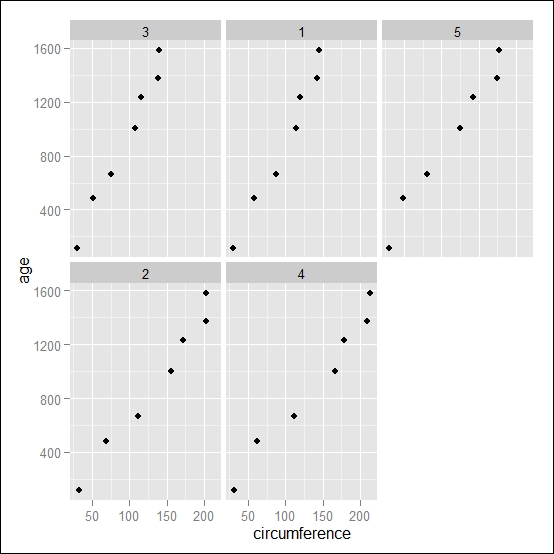### Faceting scatterplots with line and points

To plot the graph using `lattice`, use the following code:

```xyplot(age~circumference|Tree, data=Orange, type="b")
```

The preceding code results in the following output: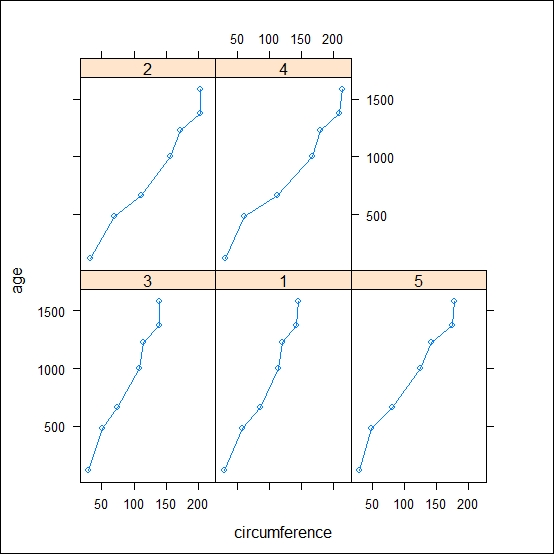To plot the graph using `ggplot2`, use the following code:

```qplot(circumference,age, data=Orange, geom=c("line","point"), facets=~Tree)
```

The preceding code results in the following output: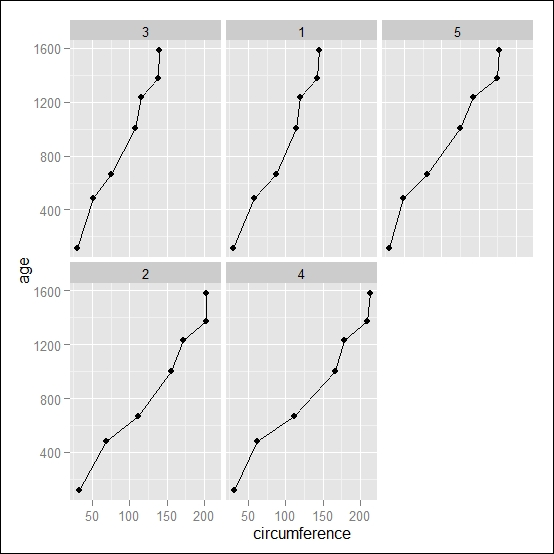### Scatterplots with grouping data

To plot the graph using `lattice`, use the following code:

```xyplot(age~circumference, data=Orange, groups=Tree, type="b")
```

The preceding code results in the following output: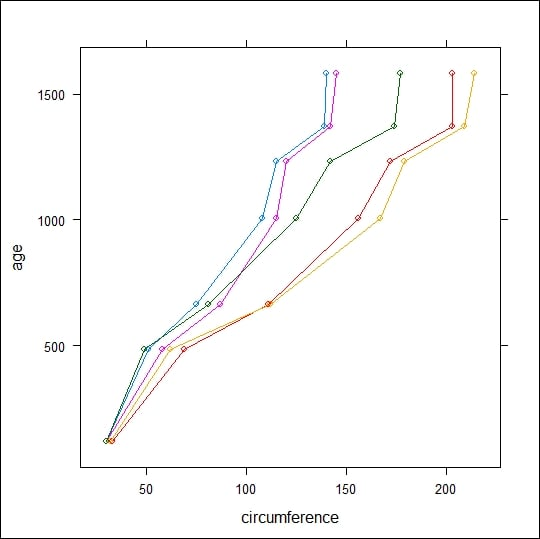To plot the graph using `ggplot2`, use the following code:

```qplot(circumference,age, data=Orange,color=Tree, geom=c("line","point"))
```

The preceding code results in the following output: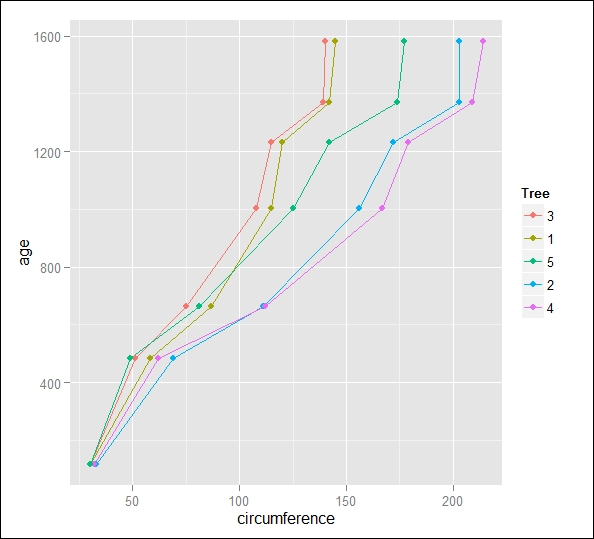### Boxplots of the orange dataset

To plot the graph using `lattice`, use the following code:

```bwplot(circumference~Tree, data=Orange)
```

The preceding code results in the following output: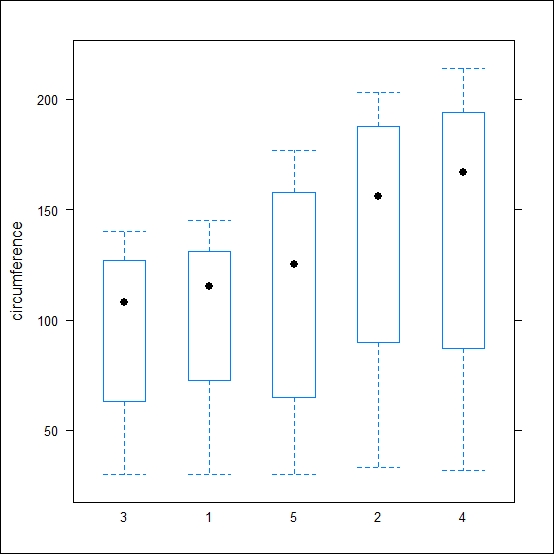To plot the graph using `ggplot2`, use the following code:

```qplot(Tree,circumference, data=Orange, geom="boxplot")
```

The preceding code results in the following output:### Histograms of the orange dataset

To plot the graph using `lattice`, use the following code:

```histogram(Orange\$circumference, type = "count")
```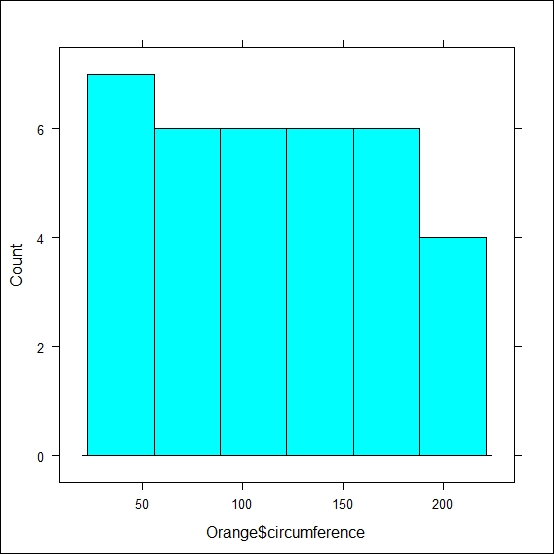To plot the graph using `ggplot2`, use the following code:

```qplot(circumference, data=Orange, geom="histogram")
```

The preceding code results in the following output:### Histograms with the reference line at the median value in red

To plot the graph using `lattice`, use the following code:

```histogram(~circumference, data=Orange, type = "count", panel=function(x,...){panel.histogram(x, ...);panel.abline(v=median(x), col="red")})
```

The preceding code results in the following output: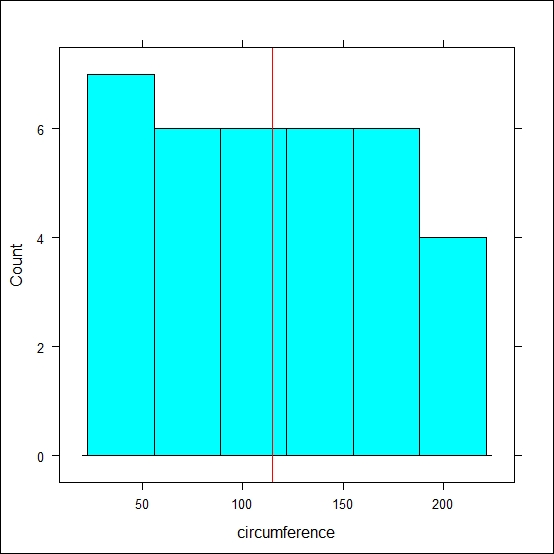To plot the graph using `ggplot2`, use the following code:

```qplot(circumference, data=Orange, geom="histogram")+geom_vline(xintercept = median(Orange\$circumference), colour="red")
```

The preceding code results in the following output:## ggplot2 and the grammar of graphics

The `ggplot2` package was developed by Hadley Wickham by implementing a completely different approach to statistical plots. As is the case with `lattice`, this package is also based on `grid`, providing a series of high-level functions that allow the creation of complete plots. The `ggplot2` package provides an interpretation and extension of the principles of the book The Grammar of Graphics by Leland Wilkinson. Briefly, The Grammar of Graphics assumes that a statistical graphic is a mapping of data to the aesthetic attributes and geometric objects used to represent data, such as points, lines, bars, and so on. Besides the aesthetic attributes, the plot can also contain statistical transformation or grouping of data. As in `lattice`, in `ggplot2`, we have the possibility of splitting data by a certain variable, obtaining a representation of each subset of data in an independent subplot; such representation in `ggplot2` is called faceting.

In a more formal way, the main components of the grammar of graphics are the data and its mapping, aesthetics, geometric objects, statistical transformations, scales, coordinates, and faceting. We will cover each one of these elements in more detail in Chapter 3, The Layers and Grammar of Graphics, but for now, consider these general principles:

• The data that must be visualized is mapped to aesthetic attributes, which define how the data should be perceived

• Geometric objects describe what is actually displayed on the plot, such as lines, points, or bars; the geometric objects basically define which kind of plot you are going to draw

• Statistical transformations are applied to the data to group them; examples of statistical transformations would be the smooth line or the regression lines of the previous examples or the binning of the histograms

• Scales represent the connection between the aesthetic spaces and the actual values that should be represented. Scales may also be used to draw legends

• Coordinates represent the coordinate system in which the data is drawn

• Faceting, which we have already mentioned, is the grouping of data in subsets defined by a value of one variable

In `ggplot2`, there are two main high-level functions capable of directly creating a plot, `qplot()`, and `ggplot()`; `qplot()` stands for quick plot, and it is a simple function that serves a purpose similar to that served by the `plot()` function in `graphics`. The `ggplot()`function, on the other hand, is a much more advanced function that allows the user to have more control of the plot layout and details. In our journey into the world of `ggplot2`, we will see some examples of `qplot()`, in particular when we go through the different kinds of graphs, but we will dig a lot deeper into `ggplot()` since this last function is more suited to advanced examples.

If you have a look at the different forums based on R programming, there is quite a bit of discussion as to which of these two functions would be more convenient to use. My general recommendation would be that it depends on the type of graph you are drawing more frequently. For simple and standard plots, where only the data should be represented and only the minor modification of standard layouts are required, the `qplot()` function will do the job. On the other hand, if you need to apply particular transformations to the data or if you would just like to keep the freedom of controlling and defining the different details of the plot layout, I would recommend that you focus on `ggplot()`. As you will see, the code between these functions is not completely different since they are both based on the same underlying philosophy, but the way in which the options are set is quite different, so if you want to adapt a plot from one function to the other, you will essentially need to rewrite your code. If you just want to focus on learning only one of them, I would definitely recommend that you learn `ggplot()`.

In the following code, you will see an example of a plot realized with `ggplot2`, where you can identify some of the components of the grammar of graphics. The example is realized with the `ggplot()` function, which allows a more direct comparison with the grammar of graphics, but coming just after the following code, you could also find the corresponding `qplot()` code useful. Both codes generate the graph depicted in Figure 1.7:

```require(ggplot2)                             ## Load ggplot2

ggplot(data=Orange,                          ## Data used
aes(x=circumference,y=age, color=Tree))+   ## Aesthetic
geom_point()+                                ## Geometry
stat_smooth(method="lm",se=FALSE)            ## Statistics

### Corresponding code with qplot()
qplot(circumference,age,data=Orange,         ## Data used
color=Tree,                                ## Aesthetic mapping
geom=c("point","smooth"),method="lm",se=FALSE)
```

This simple example can give you an idea of the role of each portion of code in a `ggplot2` graph; you have seen how the main function body creates the connection between the data and the aesthetics we are interested to represent and how, on top of this, you add the components of the plot, as in this case, we added the geometry element of points and the statistical element of regression. You can also notice how the components that need to be added to the main function call are included using the `+` sign. One more thing worth mentioning at this point is that if you run just the main body function in the `ggplot()` function, you will get an error message. This is because this call is not able to generate an actual plot. The step during which the plot is actually created is when you include the geometric attribute, which, in this case is `geom_point()`. This is perfectly in line with the grammar of graphics since, as we have seen, the geometry represents the actual connection between the data and what is represented on the plot. This is the stage where we specify that the data should be represented as points; before that, nothing was specified about which plot we were interested in drawing.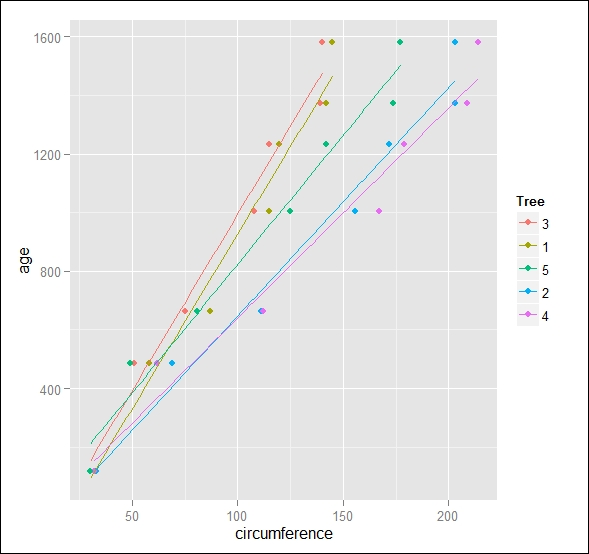Figure 1.7: This is an example of plotting the Orange dataset with ggplot2

• R Graphics (2nd edition), P. Murrell, CRC Press

• The Grammar of Graphics (Statistics and Computing) (2nd edition), L. Wilkinson, Springer

• Lattice: Multivariate Data Visualization with R (Use R!), D. Sarkar, Springer

• S-PLUS Trellis Graphics User's Manual, R. Becker and W. Cleveland, MathSoft Inc

## Summary

In this chapter, we set up your installation of R and made sure that you are ready to start creating the `ggplot2` plots. You saw the different packages available to realize plots in R and their history and relations. The `graphics` package is the first package that was developed in R; it represents a simple and effective tool to realize plots. Subsequently, the `grid` package was introduced with more advanced control of the plot elements as well as more advanced graphics functionalities. Several packages were then built on top of `grid`, in particular `lattice` and `ggplot2`, providing high-level functions for advanced data representation. In the next chapter, we will explore some important plot types that can be realized with `ggplot2`. You will also be introduced to faceting.